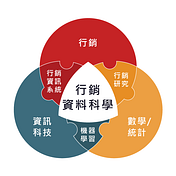# 矩陣分解推薦系統 — Python實戰：如何找出商品搭配的個體消費者策略？

`# 資料集的重新處理data = np.genfromtxt('data_core.csv', delimiter=',', names=True, dtype=(float, int, int))usuarios = data['user_id'] items  = data['item_id']ratings = data['rating'].astype(np.float32)dataset = Interactions(*(usuarios, items, ratings))`

`model = ExplicitFactorizationModel(loss='regression',#logistic                                   embedding_dim=500,                                   #120 # latent dimensionality                                   n_iter=100,                                    # 500 number of epochs of training                                   batch_size=1024,                                    # minibatch size                                   l2=1e-7,                                    # strength of L2 regularization                                   learning_rate=1e-3,                                 use_cuda=torch.cuda.is_available())from spotlight.cross_validation import random_train_test_splittrain, test = random_train_test_split(dataset, random_state=np.random.RandomState(42))print('Split into \n {} and \n {}.'.format(train, test)) # 此行產出分割的結果model.fit(train, verbose=True) #fit# save modelpickle.dump(model, open('finalized_model.sav', 'wb'))`
`train_rmse = rmse_score(model, train)test_rmse = rmse_score(model, test)`
`i = 0bought_prob = pd.DataFrame(model.predict(i), columns = ['預測利潤'])item_index = pd.read_csv('pd_index.csv')alld = pd.concat([item_index, bought_prob], axis= 1)alld['user_id'] = i`

`df2 = pd.read_csv('data_core.csv')# merge all data set togetheruser_single_data = pd.merge(df2, alld, on = ['user_id','item_id'],how='right')user_single_data['rating'] = user_single_data['rating'].fillna('沒買過')del user_single_data['item_id']user_single_data['user_id'] = user_index['user'][i]user_single_data.columns = ['利潤','會員編號','產品項目','預測利潤']# 新增推薦順序recomd = user_single_data[['產品項目','預測利潤']]recomd = recomd.sort_values('預測利潤',ascending = False).reset_index(drop = True)user_single_data['優先推薦產品'] =  recomd['產品項目']print(user_single_data)user_single_data.to_csv('user_'+ str(i) +'_recommended.csv',encoding = 'utf-8-sig')`

--

--

## More from 行銷資料科學

Marketing data science. 台灣第一個行銷資料科學(MDS)知識部落，本粉絲專頁在探討行銷資料科學之基礎概念、趨勢、新工具和實作，讓粉絲們瞭解資料科學的行銷運用，並開啟厚植數據分析能力之契機。粉絲專頁：https://www.facebook.com/MarketingDataScienceTMR

Love podcasts or audiobooks? Learn on the go with our new app.## 行銷資料科學

Marketing data science. 台灣第一個行銷資料科學(MDS)知識部落，本粉絲專頁在探討行銷資料科學之基礎概念、趨勢、新工具和實作，讓粉絲們瞭解資料科學的行銷運用，並開啟厚植數據分析能力之契機。粉絲專頁：https://www.facebook.com/MarketingDataScienceTMR